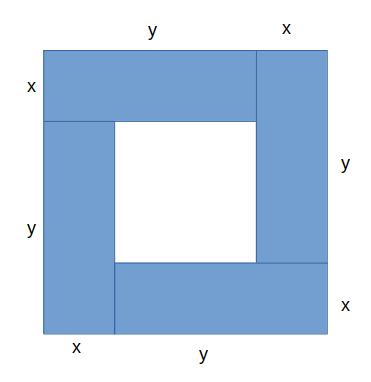Proof question: discussing with Year 12 the best method to prove $(x+y)^2 ≥ 4xy$ They can’t start with this statement, so what is your best way in to the question?

This is the sort of question where what’s useful to a student approaching the end of Year 12 might not be the same as what’s useful to a student doing Olympiad, or what’s obvious to a maths professional. There’s only one thing for it: a big laundry list of possible approaches.

## 1. Draw a picture

This is first not because it’s the most obvious starting point or even the “best” proof, but because it’s pretty, it’s cool and it’s (to me) more convincing than shuffling symbols. Here you go: the square is clearly at least as big as the four rectangles ((Without loss of generality, $x$ and $y$ can be switched))## 2. A three-stage process

This is probably the most sensible plan for a Y12 student.

• Convince yourself it’s true
• Present the case

### Convince yourself

The first step is to do exactly what the robot says you mustn’t do – on scrap paper, in the margins, somewhere away from your Actual Proper Solution, work through the algebra. For example:

• $(x+y)^2 \ge? 4xy$
• $x^2 + 2xy + y^2 \ge? 4xy$
• $x^2 - 2xy + y^2 \ge? 0$
• $(x-y)^2 \ge? 0$

Oh! Yes, yes it does. I am absolutely convinced by that. But it’s not a proof, because I’ve assumed the result.

If I wanted to do it properly, I’d need to start from something obviously true and manipulate it until I got to the proposition.

That’s easy here! I just need to start from the last line and work up the page.

### Present the case

• Consider $(x-y)^2$
• $(x-y)^2 \ge 0$
• $x^2 - 2xy + y^2 \ge 0$
• $x^2 + 2xy + y^2 \ge 4xy$
• $(x+y)^2 \ge 0 \blacksquare$

The more cynical teacher might suggest that a workable – although perhaps risky – strategy is to work up the page in the first place. You do need to be careful (working in either direction); not every conceivable algebra step is reversible, and especially when you’re multiplying/dividing by something that might be negative, or taking square roots, you need to be completely sure you’re making valid steps.

## 3. The difference approach

I like this suggestion from Pat Cobb: start from the difference between the left and right-hand sides and show that it’s non-negative.

Here, that looks like:

• Consider $f(x,y) = (x+y)^2 - 4xy$
• $f(x,y) = (x^2 + 2xy + y^2) - 4xy$
• $\dots = x^2 - 2xy + y^2$
• $\dots = (x-y)^2 \ge 0$
• So $f(x,y) \ge 0$, as required.

Now, Year 12 might not have come across proof by contradiction. However, that doesn’t make it an invalid method; on the contrary((See what I did there? Wasn’t it clever?)), I think it’s an opportunity to add tools to the mathematical toolbox.

• Assume for the purpose of contradiction that $(x+y)^2 < 4xy$
• Then $x^2 + 2xy + y^2 < 4xy$
• $x^2 - 2xy + y^2 < 0$
• $(x-y)^2 < 0$
• However, the left-hand side is the square of a real number and must be non-negative.
• We have a contradiction and our original assumption must be false((As long, as Andrew Stacey says, the law of excluded middle holds. sigh)).

## 5. Scattershot ideas

Some other approaches:

### Auxiliary variable

Let $\delta = y-x$

• Then $(x+y)^2 = x^2 + 2x(x+\delta) + (x+\delta)^2$
• $\dots = 4x^2 + 4x\delta + \delta^2$
• $\dots = 4x(x+\delta) + \delta^2$
• $\dots = 4xy + \delta^2 \ge 4xy$.

### Phantom difference of two squares

The RHS is $(2x)(2y)$, so it can be written as $(x+y)^2 - (x-y)^2$ via the difference of two squares. That’s clearly no larger than $(x+y)^2$.

### The AM-GM inequality

Now, I don’t much like this: the argument is “The AM-GM inequality states that $\frac{x+y}{2} \ge \sqrt{xy}$”, and squaring gives you the result directly. However, I feel like this is the wrong way round; I think the inequality we’re trying to prove is the reason for the AM-GM inequality, and we’re rather assuming the result. We’ve also got a problem when $xy$ is negative.

If we ignore that, though, we can use…

### A geometric proof

• A rectangle with side lengths of $x$ and $y$ has perimeter $2x+2y$.
• A square with side length of $\sqrt{xy}$ has perimeter $4\sqrt{xy}$.
• These two have the same area.
• The quadrilateral with a given area that minimises the perimeter is the square, so $2\sqrt{xy} \le x+y$
• Square both sides and draw a little square.

I would imagine there are other helpful ideas – do please share them!

* Thanks to @robotmaths, Pat Cobb, Andrew Stacey and everyone else involved in the conversation.